# Lorene::Connection Class Reference [Tensorial fields]

Class Connection. More...

#include <connection.h>

Inheritance diagram for Lorene::Connection: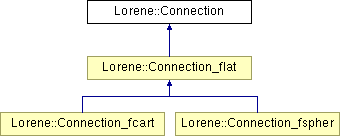List of all members.

## Public Member Functions

Connection (const Tensor_sym &delta_i, const Metric_flat &flat_met_i)
Standard constructor ab initio.
Connection (const Metric &met, const Metric_flat &flat_met_i)
Standard constructor for a connection associated with a metric.
Connection (const Connection &)
Copy constructor.
virtual ~Connection ()
Destructor.
void operator= (const Connection &)
Assignment to another Connection.
void update (const Tensor_sym &delta_i)
Update the connection when it is defined ab initio.
void update (const Metric &met)
Update the connection when it is associated with a metric.
const Mapget_mp () const
Returns the mapping.
const Tensor_symget_delta () const
Returns the tensor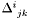which defines the connection with respect to the flat one:is the difference between the connection coefficients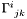and the connection coefficients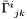of the flat connection.
virtual Tensorp_derive_cov (const Tensor &tens) const
Computes the covariant derivative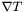of a tensor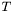(with respect to the current connection).
virtual Tensorp_divergence (const Tensor &tens) const
Computes the divergence of a tensor(with respect to the current connection).
virtual const Tensorricci () const
Computes (if not up to date) and returns the Ricci tensor associated with the current connection.

## Protected Member Functions

Connection (const Map &, const Base_vect &)
Constructor for derived classes.
void del_deriv () const
Deletes all the derived quantities.
void set_der_0x0 () const
Sets to 0x0 all the pointers on derived quantities.

## Protected Attributes

const Map *const mp
Reference mapping.
Triad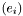with respect to which the connection coefficients are defined.
Tensor_sym delta
Tensorwhich defines the connection with respect to the flat one:is the difference between the connection coefficientsand the connection coefficientsof the flat connection.
bool assoc_metric
Indicates whether the connection is associated with a metric (in which case the Ricci tensor is symmetric, i.e.
Tensorp_ricci
Pointer of the Ricci tensor associated with the connection.

## Private Member Functions

void fait_delta (const Metric &)
Computes the differencebetween the connection coefficients and that a the flat connection in the case where the current connection is associated with a metric.

## Private Attributes

const Metric_flatflat_met
Flat metric with respect to which(member delta ) is defined.

## Detailed Description

Class Connection.

()

This class deals only with torsion-free connections.

Note that we use the MTW convention for the indices of the connection coefficients with respect to a given triad: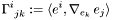Definition at line 113 of file connection.h.

## Constructor & Destructor Documentation

 Lorene::Connection::Connection ( const Tensor_sym & delta_i, const Metric_flat & flat_met_i )

Standard constructor ab initio.

Parameters:
 delta_i tensorwhich defines the connection with respect to the flat one:is the difference between the connection coefficientsand the connection coefficientsof the flat connection. The connection coefficients with respect to the triadare defined according to the MTW convention:must be symmetric with respect to the indices j and k. flat_met_i flat metric with respect to whichis defined

Definition at line 132 of file connection.C.

 Lorene::Connection::Connection ( const Metric & met, const Metric_flat & flat_met_i )

Standard constructor for a connection associated with a metric.

Parameters:
 met Metric to which the connection will be associated flat_met_i flat metric to define therepresentation of the connection

Definition at line 153 of file connection.C.

References fait_delta(), and set_der_0x0().

 Lorene::Connection::Connection ( const Connection & conn_i )

Copy constructor.

Definition at line 169 of file connection.C.

References set_der_0x0().

 Lorene::Connection::Connection ( const Map & mpi, const Base_vect & bi )  [protected]

Constructor for derived classes.

Definition at line 182 of file connection.C.

References set_der_0x0().

 Lorene::Connection::~Connection ( )  [virtual]

Destructor.

Definition at line 198 of file connection.C.

References del_deriv().

## Member Function Documentation

 void Lorene::Connection::del_deriv ( ) const [protected]

Deletes all the derived quantities.

Definition at line 208 of file connection.C.

References p_ricci, and set_der_0x0().

 void Lorene::Connection::fait_delta ( const Metric & gam )  [private]

Computes the differencebetween the connection coefficients and that a the flat connection in the case where the current connection is associated with a metric.

Definition at line 281 of file connection.C.

 const Tensor_sym& Lorene::Connection::get_delta ( ) const [inline]

Returns the tensorwhich defines the connection with respect to the flat one:is the difference between the connection coefficientsand the connection coefficientsof the flat connection.

The connection coefficients with respect to the triadare defined according to the MTW convention:Note thatis symmetric with respect to the indices j and k.

Returns:
delta}(i,j,k) =Definition at line 271 of file connection.h.

References delta.

 const Map& Lorene::Connection::get_mp ( ) const [inline]

Returns the mapping.

Definition at line 253 of file connection.h.

References mp.

 void Lorene::Connection::operator= ( const Connection & ci )

Assignment to another Connection.

Reimplemented in Lorene::Connection_flat, Lorene::Connection_fspher, and Lorene::Connection_fcart.

Definition at line 228 of file connection.C.

References del_deriv(), delta, flat_met, and triad.

 Tensor * Lorene::Connection::p_derive_cov ( const Tensor & tens ) const [virtual]

Computes the covariant derivativeof a tensor(with respect to the current connection).

The extra index (with respect to the indices of) ofis chosen to be the last one. This convention agrees with that of MTW (see Eq. (10.17) of MTW). For instance, ifis a 1-form, whose components w.r.t. the triad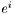are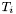: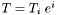, then the covariant derivative ofis the bilinear formwhose components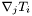are such that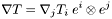Parameters:
 tens tensorReturns:
pointer on the covariant derivative; this pointer is polymorphe, i.e. it is a pointer on a Vector if the argument is a Scalar , and on a Tensor otherwise. NB: The corresponding memory is allocated by the method p_derive_cov() and must be deallocated by the user afterwards.

Reimplemented in Lorene::Connection_flat, Lorene::Connection_fspher, and Lorene::Connection_fcart.

Definition at line 310 of file connection.C.

 Tensor * Lorene::Connection::p_divergence ( const Tensor & tens ) const [virtual]

Computes the divergence of a tensor(with respect to the current connection).

The divergence is taken with respect of the last index ofwhich thus must be contravariant. For instance ifis a twice contravariant tensor, whose components w.r.t. the triad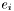are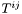: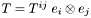, the divergence ofis the vector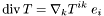where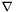denotes the current connection.

Parameters:
 tens tensorReturns:
pointer on the divergence of; this pointer is polymorphe, i.e. its is a pointer on a Scalar ifis a Vector , on a Vector ifis a tensor of valence 2, and on a Tensor otherwise. NB: The corresponding memory is allocated by the method p_divergence() and must be deallocated by the user afterwards.

Reimplemented in Lorene::Connection_flat, Lorene::Connection_fspher, and Lorene::Connection_fcart.

Definition at line 466 of file connection.C.

 const Tensor & Lorene::Connection::ricci ( ) const [virtual]

Computes (if not up to date) and returns the Ricci tensor associated with the current connection.

Reimplemented in Lorene::Connection_flat.

Definition at line 665 of file connection.C.

 void Lorene::Connection::set_der_0x0 ( ) const [protected]

Sets to 0x0 all the pointers on derived quantities.

Definition at line 216 of file connection.C.

References p_ricci.

 void Lorene::Connection::update ( const Metric & met )

Update the connection when it is associated with a metric.

Parameters:
 met Metric to which the connection is associated

Definition at line 258 of file connection.C.

References assoc_metric, del_deriv(), fait_delta(), and flat_met.

 void Lorene::Connection::update ( const Tensor_sym & delta_i )

Update the connection when it is defined ab initio.

Parameters:
 delta_i tensorwhich defines the connection with respect to the flat one:is the difference between the connection coefficientsand the connection coefficientsof the flat connection.must be symmetric with respect to the indices j and k.

Definition at line 238 of file connection.C.

## Member Data Documentation

 bool Lorene::Connection::assoc_metric [protected]

Indicates whether the connection is associated with a metric (in which case the Ricci tensor is symmetric, i.e.

the actual type of p_ricci is a Sym_tensor )

Definition at line 147 of file connection.h.

 Tensor_sym Lorene::Connection::delta [protected]

Tensorwhich defines the connection with respect to the flat one:is the difference between the connection coefficientsand the connection coefficientsof the flat connection.

The connection coefficients with respect to the triadare defined according to the MTW convention:Note thatis symmetric with respect to the indices j and k.

Definition at line 141 of file connection.h.

 const Metric_flat* Lorene::Connection::flat_met [private]

Flat metric with respect to which(member delta ) is defined.

Definition at line 156 of file connection.h.

 const Map* const Lorene::Connection::mp [protected]

Reference mapping.

Definition at line 119 of file connection.h.

 Tensor* Lorene::Connection::p_ricci [mutable, protected]

Pointer of the Ricci tensor associated with the connection.

Definition at line 164 of file connection.h.

 const Base_vect* const Lorene::Connection::triad [protected]

Triadwith respect to which the connection coefficients are defined.

Definition at line 124 of file connection.h.

The documentation for this class was generated from the following files:

Generated on 7 Dec 2019 for LORENE by1.6.1